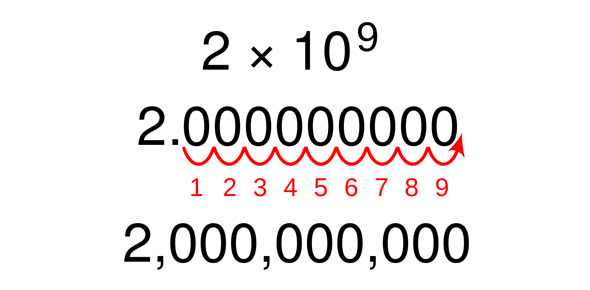# Ch. 8 Test Exponent Properties And Scientific Notation

31 Questions | Attempts: 101
ShareSettingsExponent Properties and Scientific Notationlinear systems

• 1.
Evaluate  for x = –3 and y = 2.
• 2.
Simplify 7–2.
• A.

49

• B.

–14

• C.

1/49

• D.

1/14

• 3.
Simplify –6–3
• A.

1/216

• B.

–1/216

• C.

1/18

• D.

–1/18

• 4.
Evaluate b–3 for b = 3
• A.

27

• B.

1/ 9

• C.

1/ 27

• D.

–27

• 5.
Write 2 • 10–3 as a decimal
• A.

2000 #

• B.

20,000

• C.

0.002

• D.

0.020

• 6.
Write 10–5 as a decimal
• A.

10,000

• B.

1,000,000

• C.

0.00001

• D.

0.0010

• 7.
Simplify 0.7(2.8 × 106). Write the answer using scientific notation.
• A.

19.6 × 10^6

• B.

1.96 × 10^6

• C.

19.6 × 10^5

• D.

1.96 × 10^5

• 8.
Which list has the numbers in order from least to greatest?
• A.

5.4 × 10^4, 5.4 × 10^3, 4.5 × 10^4

• B.

5.4 × 10^3, 4.5 × 10^4, 5.4 × 10^4

• C.

5.4 × 10^3, 5.4 × 10^4, 4.5 × 10^4

• D.

4.5 × 10^4, 5.4 × 10^3, 5.4 × 10^4

• 9.
Which number is written in scientific notation?
• A.

0.4 × 10^–5

• B.

68 × 10^3

• C.

12 × 10^2

• D.

5.3 × 10^–7

• 10.
Simplify 5(2.7 × 10–4). Write the answer using scientific notation.
• A.

13.5 × 10^–2

• B.

1.35 × 10^–3

• C.

13.5 × 10^–4

• D.

1.35 × 10^–5

• 11.
Simplify (0.4 × 10–6)(0.7 × 10–2). Write the answer in scientific notation.
• A.

28 × 10^–9

• B.

0.28 × 10^–8

• C.

2.8 × 10^–9

• D.

2.8 × 10^

• 12.
Simplify –3x2(6x3 + 2x)
• A.

–18x^5 – 6x^3

• B.

–6x^5 – x^3

• C.

–6x^6 – x^2

• D.

–18x^6 – 6x^2

• 13.
Simplify –4x3 • 2y–2 • 5y5 • x–8
• A.

–x to the 5th power over 40y cubed

• B.

–5y cubed over 2x to the 5th power

• C.

–2x to the 5th power over 5y cubed

• D.

–40y cubed over x to the 5th power

• 14.
. Simplify (2.3 × 10–5)(1.4 × 10–6)
• A.

3.22 × 10^–11

• B.

32.2 × 10^–12

• C.

3.7 × 10^–11

• D.

3.22 × 10^30

• 15.
Simplify (–k2)3.
• A.

# –k8 x

• B.

k^5

• C.

–k^6

• D.

–k^5

• 16.
Simplify (j5)6
• A.

j^30

• B.

J^11

• C.

j^5/6

• D.

J^15,625

• 17.
Simplify (2cd2)3(cd)6
• A.

2c^9 d^12

• B.

2c^4 d^12

• C.

8c^9 d^12

• D.

8c^9 d^8

• 18.
Simplify (11p4)–2
• A.

121p^2

• B.

1 / (121p^8)

• C.

P^2 / 121

• D.

11p^2

• 19.
Write with only one exponent.
• 20.
Simplify . Write the answer in scientific notation.
• A.

6 × 10^4

• B.

6 × 10^2

• C.

6 × 10^–4

• D.

6 × 10^3

• 21.
Simplify
• A.

D^12 / e^2

• B.

D^7/e^6

• C.

D ^12/e^8

• D.

D ^7/ e^2

• 22.
Simplify (–)–3
• A.

27/64

• B.

–27/64

• C.

–64/27

• D.

64/27

• 23.
Simplify d2(d4)5
• A.

D^18

• B.

D^11

• C.

D^22

• D.

D^13

• 24.
Chase got better at a new video game. He scored 12 points on Monday, and he doubled his score each day thereafter. How many points did he score on Sunday?
• 25.
Simplify 4–6 • 40
• A.

4096

• B.

1 / 4096

• C.

-24

• D.

0

## Related TopicsBack to top
×

Wait!
Here's an interesting quiz for you.